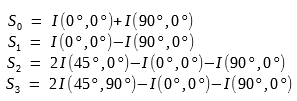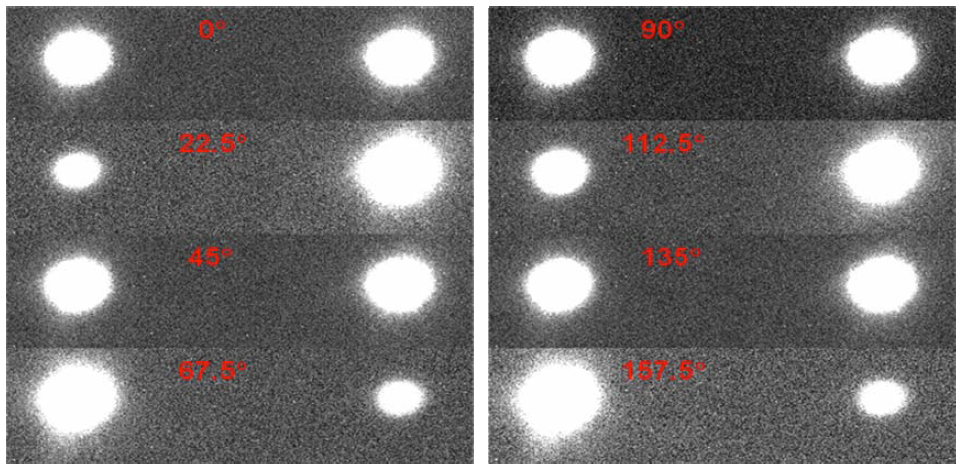# How to Measure Stokes Polarization Parameters

We will introduce the methods for measurement of Stokes polarization parameters. For the tutorial of Stokes Polarization Parameters, please check out this article.

The Stokes polarization parameters are based on intensity quantities and thus are directly measurable. This make it very useful.

The measurement is done by passing an optical beam through a retarder (waveplate) and a linear polarizer as shown below.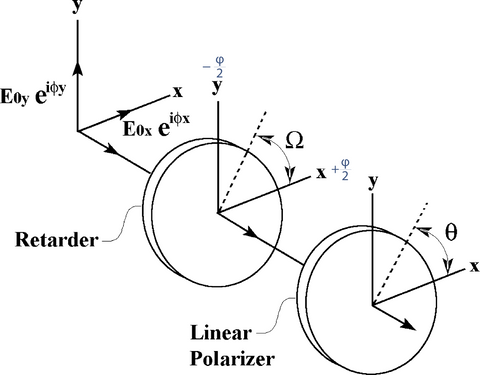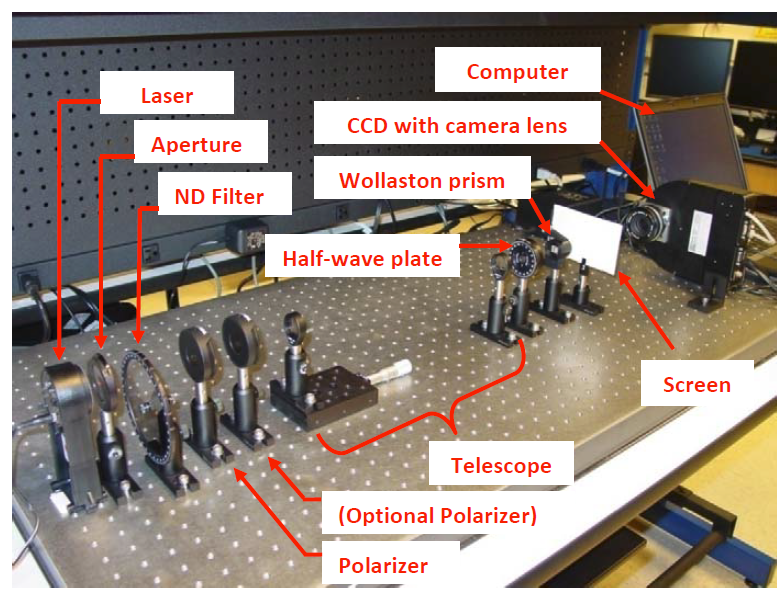A monochromatic light beam incidents on a retarder, then followed by a linear polarizer with its transmission axis aligned at an angle θ to the x axis.

We are now going to use the complex representation of the light beam, as introduced in this article.

The components of the incident beam are:where Ex and Ey are the complex amplitudes.As shown with equations (28) ~ (31) in the Stokes parameters article, the Stokes parameters for a plane wave in complex notation could be obtained from: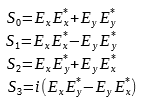where Ex* and Ey* are the complex conjugates of Ex and Ey respectively:The retarder is a phase shifting element. It can advance phase of the x component (Ex) by φ/2 ( +φ/2 ), and retard the phase of the y component Ey by φ/2 ( -φ/2 ). Thus the emerging beam after passing through the retarder is:Then, this emerging beam is incident on a linear polarizer.

The linear polarizer only allows the transmission of the optical field along an axis called its transmission axis. So only the components of Ex' and Ex' in this direction (angle θ) can transmit perfectly, while all other components are blocked as shown below.Thus, the final emerging light beam E is:And its intensity I is: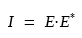After substituting E and E*, we then get the intensity I(φ,θ) which is a function of retardation angle φ and polarizer alignment angle θ:We can rewrite (5) by using trigonometric half-angle formulas, and we then get: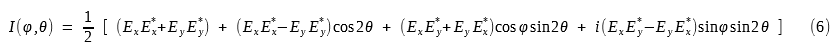Notice that the terms within parentheses are exactly the Stokes parameters given by:So we can rewrite (6) in terms of Stokes parameters and get: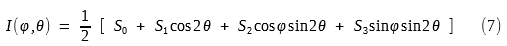This is Stokes's famous intensity formula for measuring the four Stokes parameters.

We can measure the intensities at four different pairs of φ and θ and then calculate out the four Stokes parameters as derived below.

The first three Stokes parameters S0, S1, S2 are measured by removing the retarder (waveplate) which means φ = 0°, and rotate the polarizer to the angles θ = 0°, +45°, and +90° respectively.

The final parameter S3 is measured by reinserting the quarter-wave retarder (φ = 90°) and setting θ = +45°.

The intensities are: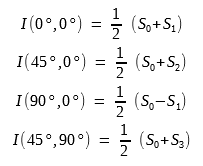We can then solve these equations and get the Stokes parameters: